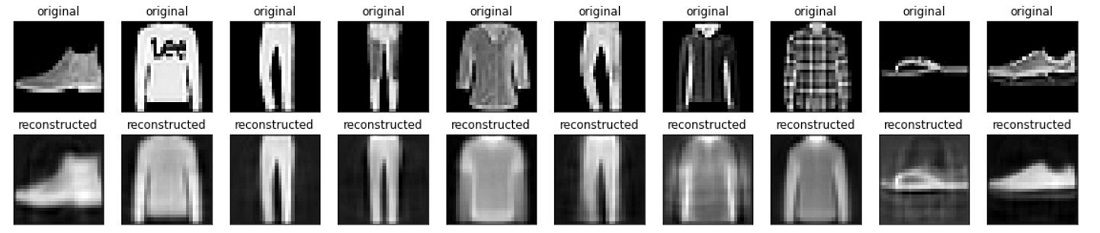# Contractive Autoencoder (CAE)

• Last Updated : 18 Jan, 2022

Contractive Autoencoder was proposed by the researchers at the University of Toronto in 2011 in the paper Contractive auto-encoders: Explicit invariance during feature extraction. The idea behind that is to make the autoencoders robust of small changes in the training dataset.

To deal with the above challenge that is posed in basic autoencoders, the authors proposed to add another penalty term to the loss function of autoencoders. We will discuss this loss function in details.

#### The Loss function:

Contractive autoencoder adds an extra term in the loss function of autoencoder, it is given as:i.e the above penalty term is the Frobinious Norm of the encoder, the frobinious norm is just a generalization of Euclidean norm.

In the above penalty term, we first need to calculate the Jacobian matrix of the hidden layer, calculating a jacobian of the hidden layer with respect to input is similar to gradient calculation. Let’s first calculate the Jacobian of hidden layer:where, \phi is non-linearity. Now, to get the jth hidden unit, we need to get the dot product of ith feature vector and the corresponding weight. For this, we need to apply the chain rule.The above method is similar to how we calculate the gradient descent, but there is one major difference, that is we take h(X) as a vector-valued function, each as a separate output. Intuitively, For example, we have 64 hidden units, then we have 64 function outputs, and so we will have a gradient vector for each of that 64 hidden unit.

Let diag(x) is the diagonal matrix, the matrix from the above derivative is as follows:Now, we place the diag(x) equation to the above equation and simplify:#### Relationship with Sparse Autoencoder

In sparse autoencoder, our goal is to have the majority of components of representation close to 0, for this to happen, they must be lying in the left saturated part of the sigmoid function, where their corresponding sigmoid value is close to 0 with a very small first derivative, which in turn leads to the very small entries in the Jacobian matrix. This leads to highly contractive mapping in the sparse autoencoder, even though this is not the goal in sparse Autoencoder.

#### Relationship with Denoising Autoencoder

The idea behind denoising autoencoder is just to increase the robustness of the encoder to the small changes in the training data which is quite similar to the motivation of Contractive Autoencoder. However, there is some difference:

• CAEs encourage robustness of representation f(x), whereas DAEs encourage robustness of reconstruction, which only partially increases the robustness of representation.
• DAE increases its robustness by stochastically training the model for the reconstruction, whereas CAE increases the robustness of the first derivative of Jacobian matrix.

## Python3

 # codeimport tensorflow as tf  class AutoEncoder(tf.keras.Model):    def __init__(self):        super(FullyConnectedAutoEncoder, self).__init__()        self.flatten_layer  =tf.keras.layers.Flatten()        self.dense1 = tf.keras.layers.Dense(64, activation=tf.nn.relu)        self.dense2 = tf.keras.layers.Dense(32, activation=tf.nn.relu)                            self.bottleneck = tf.keras.layers.Dense(16, activation=tf.nn.relu)              self.dense4 = tf.keras.layers.Dense(32, activation=tf.nn.relu)        self.dense5 = tf.keras.layers.Dense(64, activation=tf.nn.relu)                  self.dense_final = tf.keras.layers.Dense(784)                    def call(self, inp):        x_reshaped = self.flatten_layer(inp)        print(x_reshaped.shape)        x = self.dense1(x_reshaped)        x = self.dense2(x)        x = self.bottleneck(x)        x_hid= x        x = self.dense4(x)        x = self.dense5(x)        x = self.dense_final(x)        return x, x_reshaped,x_hid  # define loss function and gradientlambda =100def loss(x, x_bar, h, model):    reconstruction_loss = tf.reduce_mean(                 tf.keras.losses.mse(x, x_bar)             )     reconstruction_loss *= 28 * 28    W= tf.Variable(model.bottleneck.weights)    dh = h * (1 - h)  # N_batch x N_hidden    W = tf.transpose(W)    contractive = lambda * tf.reduce_sum(tf.linalg.matmul(dh**2 ,tf.square(W)), axis=1)    total_loss = reconstruction_loss + contractive    return total_lossdef grad(model, inputs):    with tf.GradientTape() as tape:        reconstruction, inputs_reshaped,hidden = model(inputs)        loss_value = loss(inputs_reshaped, reconstruction, hidden, model)    return loss_value, tape.gradient(loss_value, model.trainable_variables),      inputs_reshaped, reconstruction  # load dataset(x_train, _), (x_test, _) = tf.keras.datasets.fashion_mnist.load_data()x_train = x_train.astype('float32') / 255.x_test = x_test.astype('float32') / 255.# train the modelmodel = FullyConnectedAutoEncoder()optimizer = tf.optimizers.Adam(learning_rate=0.001)global_step = tf.Variable(0)num_epochs = 200batch_size = 128for epoch in range(num_epochs):    print("Epoch: ", epoch)    for x in range(0, len(x_train), batch_size):        x_inp = x_train[x : x + batch_size]        loss_value, grads, inputs_reshaped, reconstruction = grad(model, x_inp)        optimizer.apply_gradients(zip(grads, model.trainable_variables),                              global_step)              print("Step: {}, Loss: {}".format(global_step.numpy(),tf.reduce_sum(loss_value)))      # generate resultsn = 10import matplotlib.pyplot as pltplt.figure(figsize=(20, 4))for i in range(n):  # display original  ax = plt.subplot(2, n, i + 1)  plt.imshow(x_test[i])  plt.title("original")  plt.gray()  ax.get_xaxis().set_visible(False)  ax.get_yaxis().set_visible(False)    # display reconstruction  ax = plt.subplot(2, n, i + 1 + n)  reconstruction, inputs_reshaped,hidden = model(x_test[i].reshape((1,784)))  plt.imshow(reconstruction.numpy().reshape((28,28)))  plt.title("reconstructed")  plt.gray()  ax.get_xaxis().set_visible(False)  ax.get_yaxis().set_visible(False)plt.show()References:

My Personal Notes arrow_drop_up